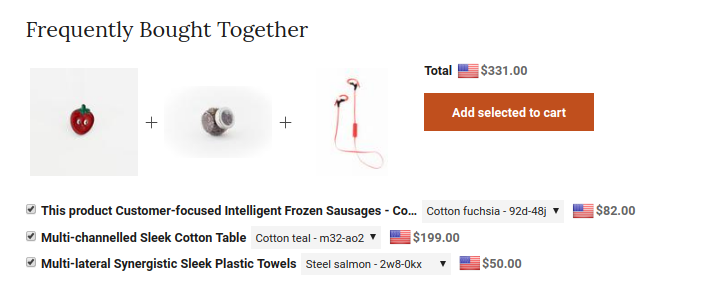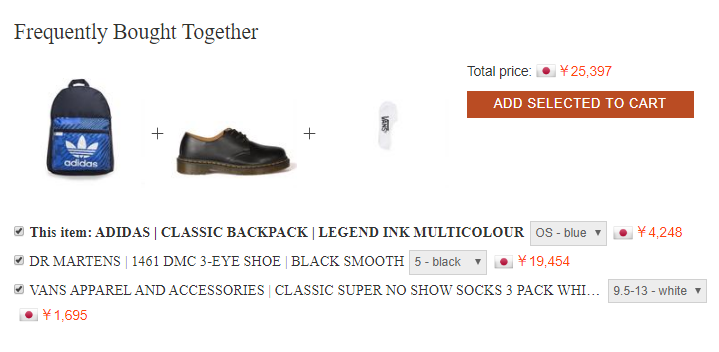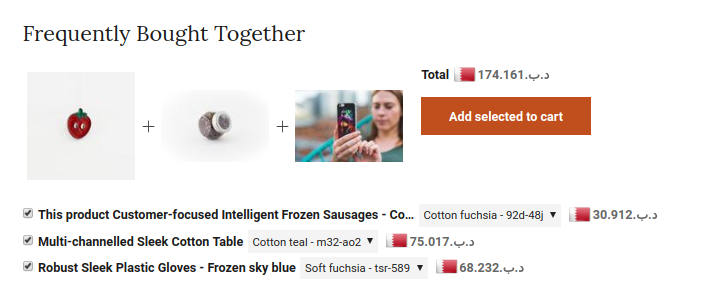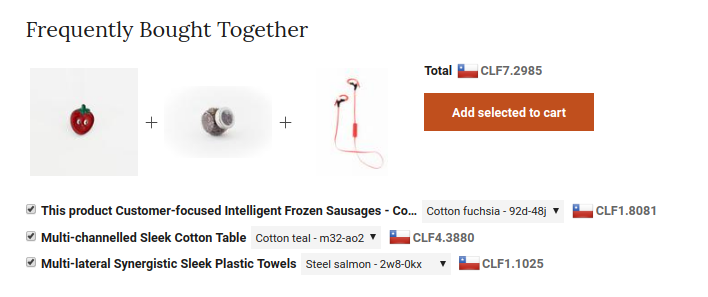# Number of decimals

The ISO 4217 standard delineates currency designators, country codes (alpha and numeric), and the number of digits after the decimal symbol. For details and more information on the matter, please check the following link https://en.wikipedia.org/wiki/ISO_4217#Active_codes.

Currency Converter + is the only currency app for Shopify that accurately displays the correct number of digits after the decimal separator according to the ISO 4217 standard.

The screenshots below show how Currency Converter+ formats the converted prices with the appropriate number of decimal digits depending on the selected currency.

#### Currency with two decimal digits

This is by far the most common situation where the converted prices show two decimal digits:#### Currency with no decimal digits

There are a number of currencies that by specification don't show decimal digits, for instance, the Japanese Yen:#### Currency with three decimal digits

For instance, the prices converted to Bahraini Dinar will show 3 decimal digits. Note that in this example, the dot in 157.000 represents the decimal symbol:#### Currency with four decimal digits

The Chilean Unit of Account (CLF) is a special case where four digits are displayed in the decimal part. Currency Converter+ also supports this currency: